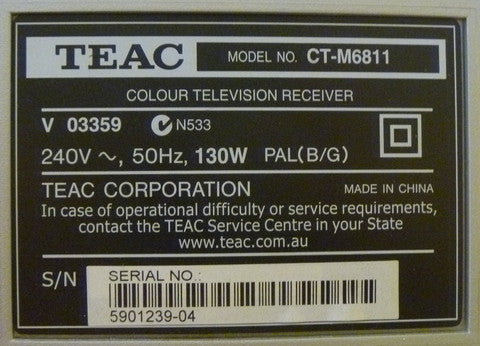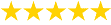# How to Calculate Wattage (Watts)

The power consumption of an appliance in watts is often said to be equal to current multiplied by voltage.

Unfortunately, it's not that simple.

This conventional wisdom or 'rule of thumb' will have you calculating Apparent Power rather than Real Power (what you get billed for).

### Apparent Power (VA)

Amps (A) x Volts (V) = Volt-Amps (VA)

The formula above can be used for calculating apparent power consumption in volt-amperes (VA). This equation will give you a rough idea of power use in watts but is not strictly correct. For this you need to take into account power factor.

### Real Power (Watts)

Amps (A) x Volts (V) x Power Factor = Watts (W)

This formula takes into account power factor, and therefore shows accurate power consumption (what you are billed for).

Confused? Use our free online electricity cost calculator.

### What is power factor?

Power Factor is a measure of the effectiveness with which an electrical device converts volt-amperes to watts. Power factor is represented as a dimensionless number between 0 and 1.

The closer the number is to one the 'better' the power factor. The higher the power factor, the more effectively electrical power is used. Resistive loads, such as most electric heaters, will have a power factor of 1 as they convert all electrical power supplied into heat. Equipment with motors, such fridges and air conditioners will have a lower power factor.

### Why is power factor important?

Power factor is important if you want to know the real power consumption of an appliance (real power is what you are billed for). See below for a demonstration of how power factor is used with our power meter to calculate the real energy consumption of my TV.

It is particularly important for larger businesses to have a power factor close to 'unity' (1) as they may be charged a fee if they have a low power factor. This is because the utility has to supply more current (amps) to the site than is actually required. In so doing, they incur more transmission losses. The good news is that businesses can take steps to increase their power factor.

### Example - calculating the actual watts of a TV

The compliance label of my TV shows the power use as 130 Watts.The problem is that compliance labels often show maximum power rather than actual power. The only way to know the actual power is to measure it with a plug-in power meter. Over a two hour period, the power meter showed a power draw of between 70 and 110 Watts - substantially less than indicated on the label.

At one point the power meter showed the TV to be using 243 volts and 0.421 Amps. If we follow conventional wisdom and just multiply Volts and Amps together without power factor, we'd work out the apparent power draw as follows:-

• Amps (A) X Volts (V) = VA
• 243 V x 0.421 A = 102.3 VA

...then falsely present it as 102.3 W

When we add power factor into the calculation, we get a very different figure. Since the power meter showed a power factor of 0.65 at that time, the calculation becomes:

• Amps (A) x Volts (V) x Power Factor = Watts (W)
• 234 V x 0.421 A x 0.65 = 66.5 W

Hopefully, you can see why it's important to get this calculation right.

Thankfully, our plug-in power meters will do these calculations for you. Be aware that some cheap power meters do not perform these measurements accurately and do not always display real power.

Our power meters display real power (Watts) as well as Amps (A), Volts (V), and power factor so you can verify the calculation if you need to.

The Reduction Revolution Plug-in Power Meter is our cheapest and most popular option. The Power Mate Lite is a high accuracy model used by professional energy auditors.

- Holly Lovell-Smith

|

### Got a Question? We're Here to Help!Read Our Customer Reviews!